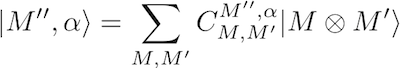# SU(N) Clebsch-Gordan coefficients

This CGC generator computes SU(N) Clebsch-Gordan coefficients (CGCs) for the decomposition of the direct product S x S' of two irreps into a direct sum of irreps S'', using a program written by Arne Alex (click to download C++ source code), based on an algorithm exploiting Gelfand-Tsetlin calculus described here [equation numbers given below in square brackets refer to this link].

## Conventions

• S = irrep label [Eq. (19)]: a sequence of N nonincreasing, nonnegative integers, the last one being 0, e.g. S = (2 2 0) for SU(3)
• dim(S) = dimension of irrep S [Eq. (22)]
• P(S) = irrep index [Eq. (C2)]: assigns to each irrep S a unique nonnegative integer
• M = state label [Eq. (20)]: a Gelfand-Tsetlin pattern, e.g. the highest-weight state of S=(2 2 0) has the form M =  2 2 0 2 2 2
• Q(M) = state index [Eq. (C7)]: assigns to each state of irrep S a unique integer between 1 and dim(S)
• alpha = outer multiplicity index [Eq. (2)]: a positive integer that distinguishes different occurences of the same irrep S'' in the decomposition of S x S'

The CGCs that express a state |M''> from irrep S'' (with outer multiplicity index alpha) as a linear combination of states |M> and |M'> from irreps S and S', respectively, are defined by the formula [Eq. (5)]:Tasks performed by the CGC-generator (1-4: decomposition of SxS'; 5-10: properties of SU(N) irreps):

S =

S' =

S =

S' =

S =

S' =

S'' =

S =

S' =

S'' =

N =

n =

S =

S =

N =

P(S) =

## 10. Find which state M is indexed by Q in irrep S

S =

Q(M) =

This page has been viewed 78662 times.